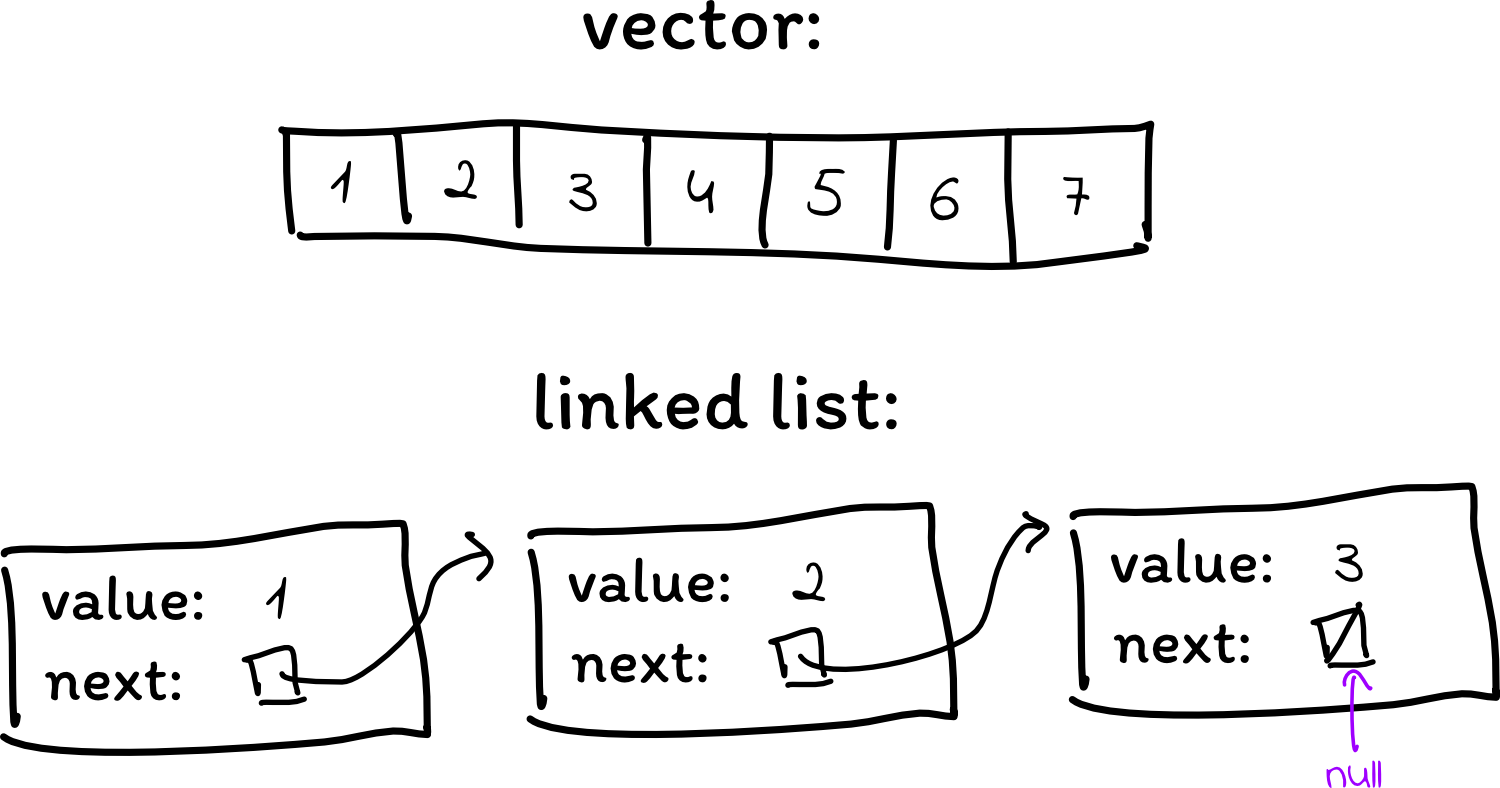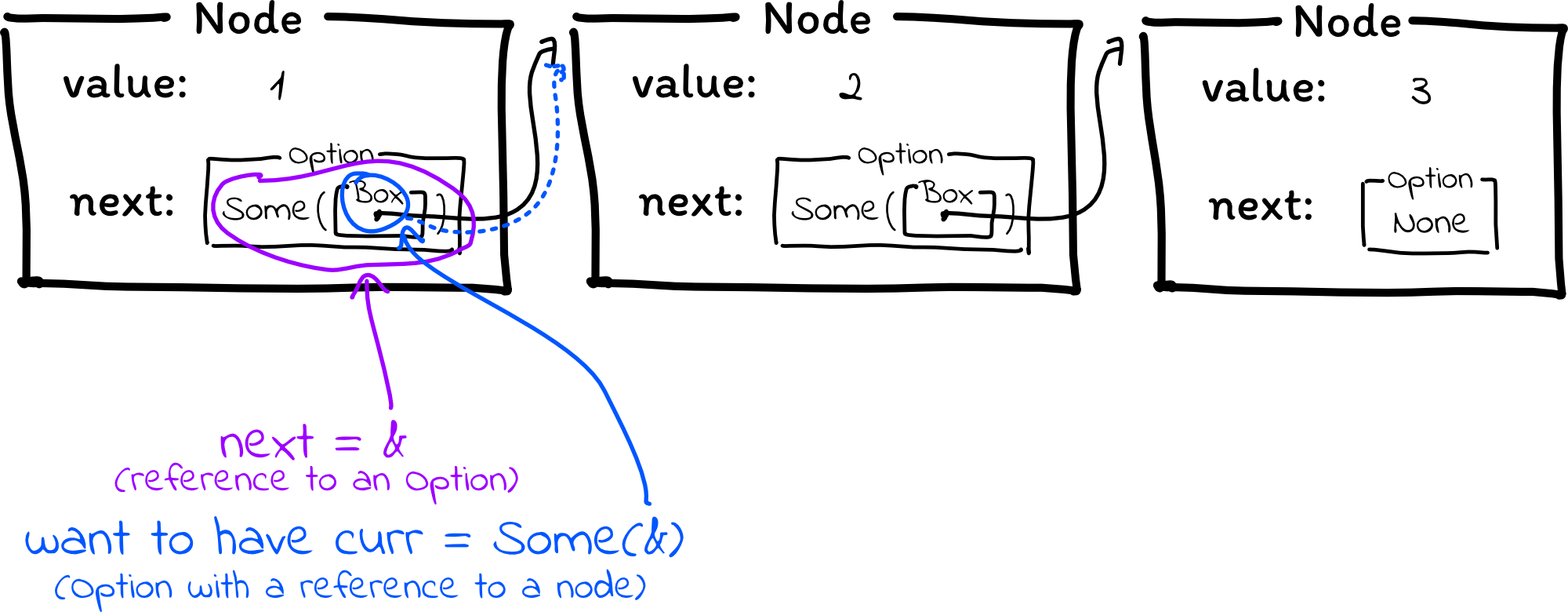# Custom Types I

## Overview

Over the course of the next two lectures, we will be implementing a linked list together in Rust! Linked lists are not all that useful in most situations, but they are very simple to understand, and their pointer-y nature will give us a challenge in Rust, helping to solidify your understanding of ownership, borrowing, lifetimes, and error handling.

## New material

Here’s a recap of new things introduced today, for anyone wanting a reference after watching the lecture:

• You can define your own types/structs using this syntax:
``````struct MyType {
field1: i32,
field2: String,
}
``````
• You can initialize a struct using this syntax:
``````let my_object = MyType {field1: 1, field2: "Hello World".to_string()};
``````
• The `Box` type stores a pointer to heap-allocated memory. You can put anything inside a `Box`; a `Box<u32>` is a heap-allocated integer (not sure why you’d do this, but you can), and a `Box<Node>` is a heap-allocated `Node`. `Box::new(...)` allocates memory and initializes it to `...`, and the `Box` drop function frees the heap memory whenever the `Box` is dropped.
• If you’re more familiar with C++, this is exactly the same as `std::unique_ptr`.
• If you have a reference to an Option with something inside (`&Option<T>`) and you want an Option containing a reference to that thing (`Option<&T>`), you can call `Option::as_ref`. This method peeks inside the Option that you have a reference to. If that Option is `None`, then it just returns you `None`. If that Option has something inside, then it peeks in, grabs a reference to that thing, and returns you a new Option that is `Some(that reference)`. You may never need to use this unless you’re implementing data structury code, and it’s totally possible to get by without it (you can do this using a 4-line `match` expression), but it’s a handy trick to have in your arsenal.

As opposed to vectors, which store data in a contiguous array, linked lists store elements in separate heap-allocated nodes, where each node points to the next node in the list.## A linked list in C

To implement this in C, we can define a node `struct` containing a value and a pointer to the next node in the list:

``````struct Node {
int value;
Node* next;
}
``````

Then, in order to create a linked list, we can allocate a few nodes, assign them values, and set their `next` pointers to point to each other:

``````int main() {
Node* first = (Node*)malloc(sizeof(Node));
first.value = 1;
Node* second = (Node*)malloc(sizeof(Node));
second.value = 2;
Node* third = (Node*)malloc(sizeof(Node));
third.value = 3;

first.next = second;
second.next = third;
third.next = NULL;
}
``````

A fun excercise might be to think about how many ways you can mess this up. I can think of several off the top of my head:

• Supplying the wrong size to `malloc`
• Forgetting to assign a `value` to one of the nodes (this will be uninitialized memory)
• Forgetting to assign a `next` pointer, particularly for the last node. If you don’t set `third.next = NULL`, then it will be an uninitialized pointer, and any code that traverses this list will follow the pointer to the next element even though no such element exists
• Forgetting to free memory, or freeing incorrectly (e.g. use-after-free)

## A linked list in Rust

First, we need to define a `Node` type. A reasonable suggestion given what you know so far is to try this:

``````struct Node {
value: i32,    // i32 is a 32-bit signed int, equivalent to "int" in C
next: Node,
}
``````

However, this won’t work because it’s an infinitely recursive type:There is no actual way to represent this in memory, since it has infinite size.

In C, we got around this problem by storing a pointer to the next node. A pointer has a fixed size of 8 bytes on 64-bit systems, so this fixes our problem. How should we do this in Rust?

So far, the closest thing we’ve seen to a pointer is a reference. However, the compiler needs to be able to analyze the code to ensure that no reference outlives the owner (in order to prevent dangling pointers / use-after-frees). Because of this, it’s usually not a good idea to put references inside of structs, because then, the compiler has a really hard time analyzing how long the reference survives, particularly if your struct gets passed around a lot.

Instead, we use something called a Box. A Box is a pointer to heap memory, where the Box constructor (`Box::new`) allocates and initializes heap memory, and the Box drop function frees the memory whenever the owner of the Box is done using it.

We can define our struct using Box like this:

``````struct Node {
value: i32,
next: Box<Node>,
}
``````## Handling nulls

This is great, but we run into problems as soon as we try creating a node. What if we want to create a list with only one node? Then `next` shouldn’t point anywhere, but Rust doesn’t have any way to create an empty Box. (That would be a null pointer, and the point of last lecture is that we want to avoid nulls.)

Instead, we use an Option to indicate whether there is a next node or not:

``````struct Node {
value: i32,
next: Option<Box<Node>>,
}
``````## Assembling a list

Now, we can create a few nodes:

``````let first = Box::new(Node {value: 1, next: None});
let second = Box::new(Node {value: 2, next: None});
let third = Box::new(Node {value: 3, next: None});
``````

And try to connect them into a list:

``````first.next = Some(second);
second.next = Some(third);
``````

This doesn’t work, but the compiler error is pretty straightforward:

``````error[E0594]: cannot assign to `first.next`, as `first` is not declared as mutable
--> src/main.rs:12:5
|
7  |     let first = Box::new(Node {value: 1, next: None});
|         ----- help: consider changing this to be mutable: `mut first`
...
12 |     first.next = Some(second);
|     ^^^^^^^^^^ cannot assign
``````

Remember that variables in Rust are constant by default. We need to add `mut` to make these mutable.

``````let mut first = Box::new(Node {value: 1, next: None});
let mut second = Box::new(Node {value: 2, next: None});
let mut third = Box::new(Node {value: 3, next: None});

first.next = Some(second);
second.next = Some(third);
``````

More errors!

``````error[E0382]: assign to part of moved value: `*second`
--> src/main.rs:12:5
|
8  |     let mut second = Box::new(Node {value: 2, next: None});
|         ---------- move occurs because `second` has type `std::boxed::Box<Node>`, which does not implement the `Copy` trait
...
11 |     first.next = Some(second);
|                       ------ value moved here
12 |     second.next = Some(third);
|     ^^^^^^^^^^^ value partially assigned here after move
``````

This is an ownership issue: the compiler is saying that `second` has been moved elsewhere, and we’re trying to use it after it has been moved.

Where did it get moved to? Let’s draw this out. When we first create the three nodes, the world looks like this:Then, after this line:

``````first.next = Some(second);
``````

the world looks like this:If you take another look at the compiler error message, you can actually see the compiler trying to explain this to us:

``````11 |     first.next = Some(second);
|                       ------ value moved here
``````

The second node has been moved out of the `second` variable and into the `Some` option that was placed in `first.next`.

This is the kind of annoying problem that happens a lot in Rust. In C, we have pointers, and we can use the pointers whenever we want (even if they aren’t actually valid anymore, which is a problem). In Rust, the compiler applies a set of specific rules to invalidate your variables whenever you transfer ownership. Although this protects us against memory errors, it can be extremely annoying, and sometimes we need to rewrite our code in different ways in order to satisfy the compiler.

Fortunately for us, there is a simple fix in our case: we can just swap the two lines.

``````second.next = Some(third);
first.next = Some(second);
``````

This first transfers ownership of the third node into the second node, then transfers ownership of the second node into the first node.Now, `first` effectively owns the entire list. When the `first` variable is no longer being used, the first `Node` will be dropped, which will cause the first `Option` to be dropped, which will cause the second Node to be dropped, and so on until all the memory is freed.

## Looping over the list

In C, the way you’d traditionally iterate over a linked list is to create a `Node*` pointer pointing to the first element; then, advance that pointer through the list, stopping once the pointer becomes NULL:

``````Node* curr = first;
while (curr != NULL) {
printf("%d\n", curr->value);
curr = curr->next;
}
``````

We can do something similar in Rust. First, we take a reference to the first element:

``````let curr: &Box<Node> = &first;
``````

Then, we can loop through until we reach the end of the list.

However, note that in the C code, we loop until `curr` is NULL, but `curr` can’t be NULL in Rust. However, we can make `curr` an `Option`, stopping the loop when we see that it is `None`:

``````let curr: Option<&Box<Node>> = Some(&first);
while curr.is_some() {
// we can unwrap the Option because we know for sure that curr is Some
println!("{}", curr.unwrap().value);
let next: &Option<Box<Node>> = &curr.unwrap().next;
curr = next;
}
``````

This code is really close, but it doesn’t quite compile because of a type mismatch. Conceptually, `next` is a reference to `curr.next`. However, `curr` is supposed to be an `Option` that is `None` if we’ve hit the end of the list, or a reference to an element in the list if we haven’t reached the end yet:There is a method called `Option::as_ref` that is handy in situations such as these. `as_ref` takes a reference to an Option. If the Option is `None`, then it returns `None` back to you, but if the option is `Some`, then it peeks inside and returns to you `Some(a reference to the thing inside)`. It’s not often that you need this unless you’re working with data structure-y code, but it can be handy to keep in your bag of tricks.

``````let curr: Option<&Box<Node>> = Some(&first);
while curr.is_some() {
// we can unwrap the Option because we know for sure that curr is Some
println!("{}", curr.unwrap().value);
let next: &Option<Box<Node>> = &curr.unwrap().next;
// Get an Option with a reference to the next element
curr = next.as_ref();
}
``````

And with that, we have a linked list that we can iterate over and print out!